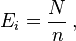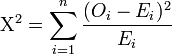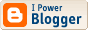## T-SQL: Generating Random Numbers, Random Sampling and Random ‘Goodness’

Note: I will use the word ‘random’ throughout to mean ‘pseudo-random’. If you want truly random numbers then you need to sample a physical source of noise, i.e. you need one of these devices: http://www.idquantique.com/true-random-number-generator/products-overview.html !

If you are working with large volumes of data, it’s a common requirement to select a smaller, random sample of that data. There are several techniques to do this using TSQL; we will examine several, and perform a statistical evaluation of the distribution of the samples that each technique produces.

There are two common scenarios. Consider a data warehouse that contains hundreds (or thousands) of millions of rows. Working with the entire dataset to perform statistical analysis or data mining can be very time-consuming.  Instead, analysts often select a smaller, random sample of records to build a model or draw conclusions, and then test these predictions against the full population.

Alternatively, analysts could use a BI tool (such as OLAP or data mining) to study the entire dataset and identify patterns that might represent a business opportunity. By testing against small random subsets of data, analysts can verify that their hypotheses hold true.

If you search the internet for “TSQL Random Sampling” most paths lead back to this article “Random Sampling in T-SQL”, written by Brian Connolly back in 2004. As the code no longer appears to be available in its entirety, I’ve rewritten the code but followed some of the same techniques used in that article.

There are several techniques that can be used to implement random sampling with TSQL:

• Add a column to the table, fill it with values from the Rand() function, and select an ordered subset based on that column.
• The same as the first technique, except that the Rand() function is seeded.
• The same as the first technique, except that the Rand() function is anchored to a row.
• Use the NEWID() GUID function to select an ordered subset.
• Use CHECKSUM(NEWID()) as a source of random values.
• Use TABLESAMPLE introduced in SQL Server 2008.

Before going any further, we can quickly rule out limiting result sets using TABLESAMPLE:

You can use TABLESAMPLE to quickly return a sample from a large table when either of the following conditions is true:

• The sample does not have to be a truly random sample at the level of individual rows.
• Rows on individual pages of the table are not correlated with other rows on the same page.

In other words, despite its name, if you want a truly random sample don’t use the built-in TABLESAMPLE!

Some considerations:  Rand() is relatively expensive because it requires the use of INSERTs, cursors, or single-row UPDATEs. Seeded Rand() and NewID() are easier to use, but are they sufficiently random?  What we want to determine is the quality of the statistical random samples they produce. There are many statistical tests to determine the randomness of a pseudorandom number generator, which we expect to have a uniform distribution. One of the most commonly used is the Chi-square Test:

###### Discrete uniform distribution

In this case, N observations are divided among n cells (or buckets). A simple application is to test the hypothesis that, in the general population, values would occur in each cell with equal frequency. The "theoretical frequency" for any cell (under the null hypothesis of a discrete uniform distribution) is thus calculated as###### Calculating the Chi-Square test-statistic

The value of the test-statistic iswhere

• Χ2 = Pearson's cumulative test statistic, which asymptotically approaches a χ2 distribution.
• Oi = the observed frequency;
• Ei = the expected (theoretical) frequency, asserted by the null hypothesis;
• n = the number of buckets that the sample is divided into.

The Chi-squared test divides the row range into a number of equally spaced "buckets" and ensures that on average the number of items in each bucket falls within the expected count (which for a uniform distribution is simply the number of rows divided by the number of buckets). For example, a random 1 percent sample of a RandomPopulation table with 100,000 rows will return 1000 row ids that are scattered between 1 and 100,000. Divide the row space into 40 "buckets" from 1-2500, 2501-5000 ... 97501-100000. A random 1 percent sample from RandomPopulation should place roughly 25 row ids into each bucket (some buckets will have more and some will have less). The Chi-square test measures this deviation to determine how much non-randomness is present.

#### First Attempt Using Rand()

So you have a problem where you need to select some random sampled data in TSQL, and therefore need a source of ‘random’ numbers. Your first attempt at generating random numbers might be something along the lines of:

   -- Won't work: returns same value for all rows!   SELECT TOP 1000    RAND() AS rnd   FROM master.sys.all_columns AS ac1   CROSS JOIN master.sys.all_columns AS ac2

But you will quickly discover that a major drawback to using Rand() without some form of ‘row anchor’ is that it is only evaluated once per query. So the previous query returns the same random number repeated for each row.

#### The RandomPopulation table

Following the original implementation, we will use a similar table to implement and compare these techniques:

   create table dbo.RandomPopulation   (       rowid int not null PRIMARY KEY,      pure_random float null,      seeded_random float null,      seeded_random_anchored float null,      newid_random float null,      newid_guid UNIQUEIDENTIFIER null,      pure_random_order int null,      seeded_random_order int null,      seeded_random_anchored_order int null,      newid_random_order int  null,      newid_guid_order int null   )

This table will contain the population to be sampled. The first group of columns hold the actual random values generated, while the second group hold rowid values when ordered by their respective random value column.

If there are 100,000 rows in the table, rowid will have values between 1 and 100,000 inclusive. The row with the smallest pure_random number will have a pure_random_order value of 1, and similarly the row with the largest pure_random number will have a pure_random_order value of 100,000. We use queries like the following to return a sample of RandomPopulation with @samplecount rows into a temporary table:

   select rowid   into #tpure   from RandomPopulation   where pure_random_order < @rowsample

The other random value columns are processed similarly.

#### Method 1: Fill a column using rand() in a while loop

Since the only way to assign a different random number to every row in a table is to make a separate assignment for each row, one solution is to  loop over the rows, updating the table one row at a time:

   -- 1. Pure random value   set @row = 1   while (@row <= @rowcount)   begin      insert into dbo.RandomPopulation (rowid, pure_random)      values (@row, rand())      set @row = @row + 1   endOnce all rows have been assigned a pure_random value, the pure_random_order column can be calculated:

   update dbo.RandomPopulation   set pure_random_order = x.row_order   from RandomPopulation    inner join (select row_number() over(order by pure_random) as row_order, rowid                from dbo.RandomPopulation) x on  x.rowid = dbo.RandomPopulation.rowid

This query pattern is likewise repeated for the other random value/order column pairs.

#### Method 2 and 4: rand() seeded with Newid(), and pure Newid()

In order for rand() to be evaluated for each row, we need to seed it with a row-dependent value. TSQL’s NewID() function is evaluated once per row, and the uniqueidentifier values (128 bit GUIDs) that it returns can be ordered. Both of these methods are shown below:

   -- 2. Rand seeded with guid     UPDATE dbo.RandomPopulation    SET seeded_random = rand(checksum(newid()))
   -- 4. newid() value   UPDATE dbo.RandomPopulation    SET newid_guid = newid()

#### Method 3: rand() seeded with a row anchor

As mentioned earlier, if rand() is seeded with a row-dependent value, it's evaluated not once per query, but rather once for each row. In Brian’s original article, he uses the following formula to seed rand() with a row-dependent value:

   -- 3. rand seeded and anchored to row: DO NOT USE!!   UPDATE dbo.RandomPopulation    SET seeded_random_anchored = rand((rowid % 1000000) * (datepart(ms, getdate())+1))

This formula does not work at all well. You do not even need to perform a chi-square test on the data ; it is visibly predictable!

Instead, I used the following formula:

   -- 3. rand seeded and anchored to row (2): also, DO NOT USE!!   UPDATE dbo.RandomPopulation   SET seeded_random_anchored = rand((rowid % 97) * (datepart(nanosecond, sysdatetime())/100 + 1))

The seed chosen for rand() is a function of the rowid and also the current nanoseconds. This is the one I’ve included in the results, BUT both versions perform very poorly, and should not be used in practice. Here’s what Brian had to say:

The use of seeded random is problematic. Rand has been designed (and tested) to give a stream of statistically independent, uniformly distributed numbers on successive calls after it's seeded. However, the "seeded random" technique used earlier essentially takes the first random number from a different seed for each row. So while Rand(X), Rand(), Rand() ... Rand() would be expected to yield a valid stream of random numbers, there's no reason to expect Rand(N1), Rand(N2) ... Rand(NNN) to be random. For this reason, and also because the tests that follow demonstrate how bad it can be, I don't recommend you use this technique.

Although using a time based function to seed rand() might seem convenient, in addition to the above reasons for not doing so, there is also the issue that the ‘randomness’ is affected by the speed of your CPU!

#### Method 5: checksum(newid) as a float value

This method transforms the 128bit GUID returned from Newid() into a 32bit integer value using Checksum() and then into a floating point value between 0 and 1.

   -- 5. checksum(newid()) value   UPDATE dbo.RandomPopulation    SET newid_random = CAST(CHECKSUM(NEWID()) & 0x7fffffff AS float) / CAST (0x7fffffff AS int)

The expression CAST(CHECKSUM(NEWID()) & 0x7fffffff AS float / CAST (0x7fffffff AS int) evaluates to a random float value between 0 and 1.  The idea behind testing this is to check whether the restriction of the GUID values to 32 bits still renders them sufficiently random.

Note: This technique can be used directly to select a random sample of individual rows. In this example from MSDN, the following query uses the NEWID function to return approximately one percent of the rows of the Sales.SalesOrderDetail table:

   SELECT * FROM Sales.SalesOrderDetail   WHERE 0.01 >= CAST(CHECKSUM(NEWID(), SalesOrderID) & 0x7fffffff AS float) / CAST (0x7fffffff AS int)

The SalesOrderID column is included in the CHECKSUM expression so that NEWID() evaluates once per row to achieve sampling on a per-row basis. The expression CAST(CHECKSUM(NEWID(), SalesOrderID) & 0x7fffffff AS float / CAST (0x7fffffff AS int) evaluates to a random float value between 0 and 1.

-------------------------------

Sidebar: Picking a single random row

Picking a single random row using an ORDER BY clause like this:

   SELECT TOP 1 * FROM table ORDER BY CHECKSUM(NEWID())

performs a table scan (because the random value associated with each row obviously needs to be calculated before the rows can be ordered), which can be slow for large tables. Using an indexed integer column (such as that commonly used for a primary key), and using:

   SELECT TOP 1 * FROM table    WHERE rowid >= RAND(CHECKSUM(NEWID())) * (SELECT MAX(rowid) FROM table)

works in constant time, provided the rowid column is indexed. Note: this assumes that rowid is uniformly distributed in the range 0..MAX(rowid). If your dataset has some other distribution, your results will be skewed (i.e. some rows will be picked more often than others).

-------------------------------

#### Running the Tests

I have followed Brian’s original TSQL implementation to calculate the bucket frequencies and the resulting chi-square statistic. Please refer to the original article or the attached downloadable code for more details. The tests are wrapped in the following stored procedure (available in the download):

create procedure dbo.test_random_selection(   @runs        int,        -- Number of times to run the tests   @rowcount    int,        -- Size of the Random population to be sampled   @sampleprop  float,      -- Proportion of the Random population to be sampled   @bucketcount int         -- Number of buckets to be used for chi-square analysis)

#### Test Results

All tests were run against SQL Server 2008 R2 SP1 Enterprise Edition (x64) (and also without SP1; the results were similar) on an Intel Quad core i7 based PC running Windows 7 x64 Ultimate.

-------------------

Test1: test_random_selection(50, 100000, 0.01, 40): 50 runs, 100,000 RandomPopulation rows, a 1 percent sample, and 40 buckets.

Each 2,500 (= 100,000 / 40) interval range bucket should contain approximately 25 rowids. There are 39 degrees of freedom (1 less than the number of buckets). We can say that the row sample for each technique is independent and uniformly distributed at the 90% confidence level if the Chi-square statistic for each technique is Chi-Sq[.90, 39]. Consulting a standard Chi-square table gives a value of 50.660 for the Chi-Sq[.90, 39] threshold level of this statistic (and the lower Chi-Sq[.10, 39] = 28.196). As long as the Chi-square value for each technique is lower than 50.66, there is no reason to suspect that the sample is not random (Note: we expect that some samples will exceed the threshold value, because we are working with a confidence level of 90%. A few instances of crossing this value does not necessarily mean that the samples are non-random).

The results from this test are shown here:Rand() Rand(Seeded) Rand(anchored) Checksum(Newid()) Newid Avg 38.12 38.90 23.26 38.38 38.67 Std 10.18 8.73 2.92 9.17 9.40 Min 19.64 23.64 18.44 21.08 18.20 Max 68.68 60.64 26.88 60.68 57.60 # >= 50.66 6 5 0 4 6

The Rand() seeded with a row anchor technique is showing very predictable behaviour. In fact, it is producing an unusual number of equal values!. The averages, standard deviations, max and min values for the other techniques are very similar to each other in shape and bounds.

Out of interest, I ran the tests again using the rand anchored formula from the original article, and obtained a similar result to Brian:

Neither of the ‘rand() seeded with a row anchor’ methods produce anything like sufficiently random sample, and consequently should not be used in practice.

Test 2: test_random_selection(160, 1000000, 0.01, 100): 160 runs, 1,000,000 RandomPopulation rows, a 1 percent sample, and 100 buckets.

Consulting a standard Chi-square table gives a value of 117.407 for the Chi-Sq[.90, 99] threshold level of this statistic (and the lower Chi-Sq[.10, 99] = 81.449). I have omitted the seeded anchored results in the larger graph to improve the viewable scale (but have included with the same results below, reduced in size)Rand() Rand(Seeded) Checksum(Newid()) Newid() Avg 99.17 99.06 95.75 97.20 Std 14.27 15.14 13.38 14.7 Min 69.27 69.72 63.92 70.41 Max 138.11 145.07 131.39 138.57 # > 117.407 16 23 7 14 # < 81.449 15 21 27 21

#### Conclusions

The seeded random anchored technique should never be used: the results were inconsistent and generally very poor.

The other 4 random sampling techniques produced very similar, acceptable results. They produced random samples that were sufficiently random as measured by a Chi-Square test. rand() has been designed to meet statistical standards so it is no surprise it produces random numbers whose distribution is uniform and satisfy a Chi-Square test. You should use rand() wherever possible, but bear in mind the overhead it has when used as a ‘row at a time’ operation. In testing, I found generating rand() per row was 60 times slower than the set-based operation of either using checksum(newid()) or even rand(checksum(newid())):

Filling a table column for 1 million rows:

Rand() per row                      : 124 seconds
Set:CHECKSUM(NEWID())       : 2 seconds
Set:rand(checksum(newid())) : 2 seconds

So, if speed is a factor, then consider using of the newid()techniques. But heed Brian’s advice:

Bear in mind, though, that because NewID() is assigned by the operating system, you should test its behaviour on the target system–perhaps using the code included in the accompanying Download as a starting point.

#### Notes:

Note: none of the random number generation methods discussed here are sufficient for cryptographic purposes (which require much larger number generation spaces). SQL Server 2008 introduced the CRYPT_GEN_RANDOM(length [, seed ]) function, which returns a cryptographic random number generated by the Crypto API (CAPI). The output is a hexadecimal number of the specified number of bytes.

Note: Please be aware of the following issue, when using Newid() or any other non-deterministic function in a table expression (derived table, CTE, view, inline TVF):

#### Refs:

RandomNoTest.zip

Mitch, I thought I'd return the favor and check out your blog. (I saw the link this morning for the first time.) I'm bowled over by the random number article. So well written, so thorough. I happen to be working on a project that's using them. I'll be sure to go through this more carefully. Terrific stuff. Sincerely, Duffy

ByMichael Duffy, at August 18, 2011 6:08 pm

<< Home###### Contact

MSN, Email: mitch døt wheat at gmail.com